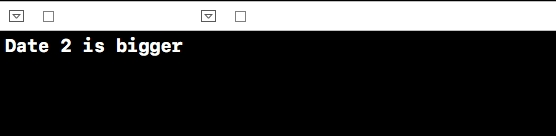# How to compare two NSDates in iPhone/iOS?

In this article we'll see how to compare two NSDates in swift. First of all we'll need to create two NSDates.

We'll do it in playground instead of simulator this time.

First Let's create two different dates.

let dateOne = NSDateComponents()
dateOne.day = 5
dateOne.month = 6
dateOne.year = 1993
let dateTwo = NSDateComponents()
dateTwo.day = 4
dateTwo.month = 2
dateTwo.year = 1995

Using these date components we'll create dates and then compare them

let cal = NSCalendar.current
let FirstDate = cal.date(from: dateOne as DateComponents)
let secondDate = cal.date(from: dateTwo as DateComponents)

Now to compare them we'll use a if condition.

if secondDate!.compare(firstDate!) == .orderedAscending {
print("date 1 is bigger than date 2")
} else {
print("Date 2 is bigger")
}

Below is the output of above code when running on simulator.there are three different ways we can compare.

• orderedAscending
• orderedDescending
• orderedSame